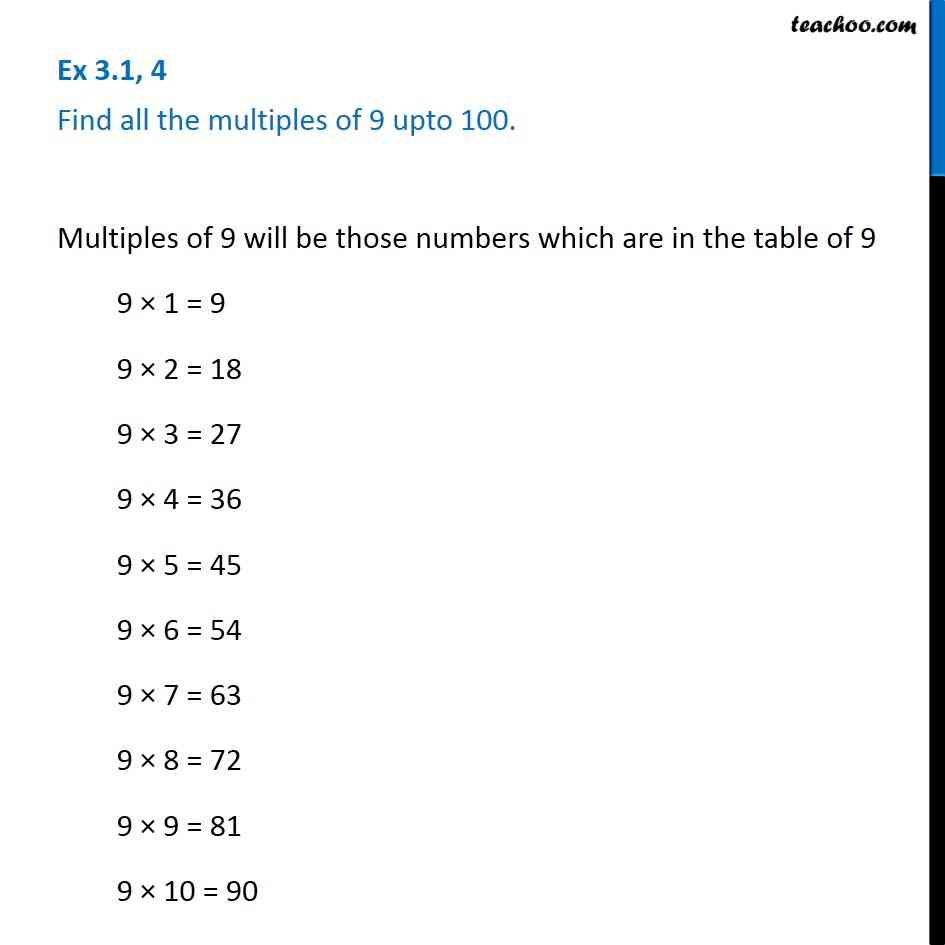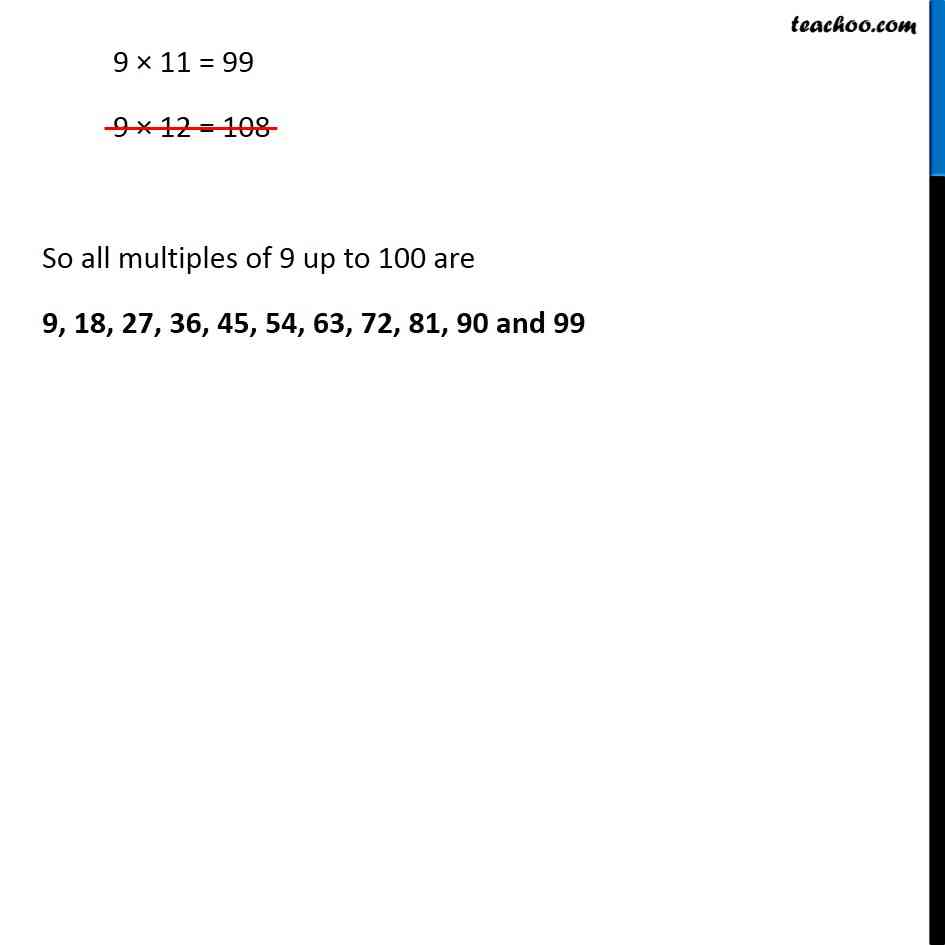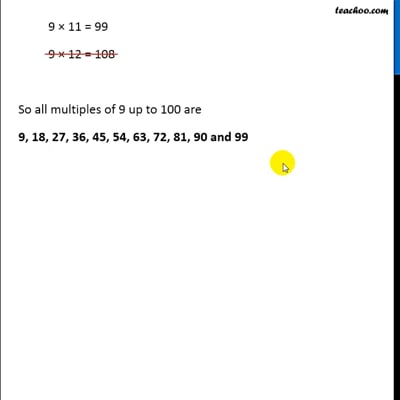Factors and Multiples

Chapter 3 Class 6 Playing with Numbers
Concept wiseThis video is only available for Teachoo black users

Introducing your new favourite teacher - Teachoo Black, at only ₹83 per month

### Transcript

Ex 3.1, 4 Find all the multiples of 9 upto 100. Multiples of 9 will be those numbers which are in the table of 9 9 × 1 = 9 9 × 2 = 18 9 × 3 = 27 9 × 4 = 36 9 × 5 = 45 9 × 6 = 54 9 × 7 = 63 9 × 8 = 72 9 × 9 = 81 9 × 10 = 90 9 × 11 = 99 9 × 12 = 108 So all multiples of 9 up to 100 are 9, 18, 27, 36, 45, 54, 63, 72, 81, 90 and 99